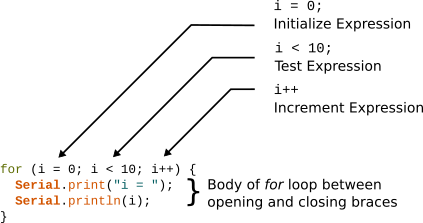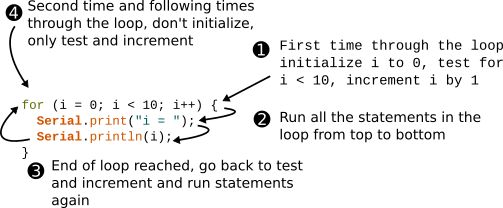Starting Electronics Needs Your Help!
It is that time of the year when we need to pay for web hosting and buy new components and equipment for new tutorials. You can help by making a donation. Contribute to this website by clicking the Donate button. The total will be updated once daily. (You may need to clear your browser cache to see the updates.)Target Amount: \$2000
Amount Raised: \$1579.10

Top Donor: C.C. \$100

X

# The Arduino for Loop

Created on: 1 October 2014 | Updated on: 23 January 2017

Part 7 of the Arduino Programming Course

We have already looked at one type of loop on this course namely, the Arduino main loop in part 2.

In this part of the Arduino programming course, we look at another kind of loop called the "for" loop.

Whereas statements or code in the Arduino main loop will run continually and never exit the loop, the for loop allows us to loop through code a certain number of times before exiting the loop.

A common way to use the for loop is with the increment operator that was covered in the previous part of this course.

## Using the for Loop

The following sketch demonstrates the use of the for loop.

```void setup() {
int i;

Serial.begin(9600);

for (i = 0; i < 10; i++) {
Serial.print("i = ");
Serial.println(i);
}
}

void loop() {
}
```

Load the sketch to your Arduino to see how it runs. This video shows the sketch running.

Can't see the video? View on YouTube →

### How the for Loop Works

The image below shows the parts of the for loop.Parts of a for Loop in an Arduino Sketch

#### for Loop Structure

A basic for loop is started as follows:

```for () {
}
```

Three expressions are added between the opening and closing parentheses () that determine how many times the statements in the loop are run before exiting the loop. When the loop is exited, program execution continues below the loop – i.e. statements outside and below the loop are executed from top to bottom.

The body of the loop between the opening and closing braces {} contains statements that will run in the loop.

The expressions between the parentheses are called the initialize expression, test expression and increment expression.

A variable must be defined to use in the three loop expressions. In the example sketch an integer variable called i is used.

The three loop expressions must appear in the order: initialize, test and increment. They are separated by semicolons (;). The increment expression does not end with a semicolon.

#### Initialize Expression

The initialize expression is only run once at the time that the loop starts. It initializes our i variable to zero (0) in the example sketch.

#### Test Expression

The test expression determines when we break out of the loop. It is used to set the number of times that the statements in the body of the loop are run.

When the test expression evaluates to true, the statements in the loop body will be run. When the test expression evaluates to false the loop will not be run again, but will be exited.

The test expression is evaluated every time that execution starts at the top of the loop.

#### Increment Expression

The increment expression is used to change the value that the i variable holds. It is run every time that execution starts at the top of the loop.

#### Program Flow in the Loop

The image below shows how program flow works in the for loop.for Loop Program Flow

#### First Time Through the Loop

The first time through the loop, i is initialized to 0, the test expression tests whether i < 10 (0 < 10) which is true, so the statements in the loop will run.

Because the post increment operator is used with the variable, i will only be incremented at the end of the loop. The statements in the loop run and print the value of i as 0 because it has not yet been incremented.

We therefore have this:

```i is initialized to 0
i contains 0
i < 10 evaluates to true or 1 because i is less than 10
The two statements in the loop run, print i as 0
At the end of the loop i is incremented so i == 1
```

#### Second Time Through the Loop

The second time through the loop, i now contains 1 as it was incremented at the bottom of the loop. The test expression now tests whether i < 10 (1 < 10) which is true, so the statements in the loop will run again. The i variable will only be incremented to 2 at the bottom of the loop, so 1 is printed to the serial monitor window.

We now have this:

```i is not initialized again
i contains 1
i < 10 evaluates to true or 1 because i is less than 10
The two statements in the loop run, print i as 1
At the end of the loop i is incremented so i == 2
```

#### Last Time Through the Loop

Execution of the loop will continue and i will be incremented every time.

The last time through the loop, we have this:

```i is not initialized again
i contains 9
i < 10 evaluates to true or 1 because i is less than 10
The two statements in the loop run, print i as 9
At the end of the loop i is incremented so i == 10
```

Execution starts at the top of the loop again, the evaluation expression is tested.

We now have this:

```i is not initialized again
i contains 10
i < 10 evaluates to false or 0 because i is not less than 10 (it is equal to 10)
The statements in the loop are not run again
The loop is exited
The statement below the closing bracket of the loop will be run
```

Amazon.com

Amazon.co.uk

### Alternative Way of Writing the for Loop

The sketch that follows shows that the variable used in the loop expressions can also be defined in the loop and does not have to be defined outside the loop as the previous sketch did.

```void setup() {
Serial.begin(9600);

for (int i = 0; i < 10; i++) {
Serial.print("i = ");
Serial.println(i);
}
}

void loop() {
}
```

This sketch works exactly the same as the previous sketch and outputs the numbers 0 to 9.

## A Loop Within a Loop

The next sketch uses a for loop within the Arduino main loop.

```void setup() {
Serial.begin(9600);
}

void loop() {
for (int i = 0; i < 10; i++) {
Serial.print("i = ");
Serial.println(i);
}
delay(1000);
}
```

The for loop works exactly the same as it did before, but now after it has been exited, the delay() function is run to give a 1 second delay. The end of the Arduino main loop loop() is reached, so the for loop is run again.

When the for loop is run again, i is initialized to 0 because the for loop is being started from the top again. It then runs again as previously described.

The for loop and delay() function will be run continually because the main Arduino loop never exits.

## Notes on the for Loop Sketch Examples

Note the following about the sketch examples in this part of the course.

### Initialize Expression

The initialize expression in the for loop does not have to be initialized to zero (0), but can be initialized to any integer value, even a negative value.

### Increment Expression

The increment expression is used to change the value of the variable that the test expression tests. This does not have to be an increment operator, but can be the decrement operator (subtracts 1 from the variable) or any other arithmetic expression.

The increment operator has been used in the example sketches to keep things simple at the beginning of the course, and because it is a common way of using the for loop. We will look at other ways to use the for loop later in the course.

### Counting from Zero (0)

Note that in the example sketches, that the value that the i variable contains is initialized to 0 and not 1. We therefore print out a count value that starts at 0 and ends at 9.

The loop is actually run 10 times and not 9 times. This is because we are starting at 0 – 0 to 9 are 10 numbers, 1 to 9 are 9 numbers, 1 to 10 are 10 numbers.

This list shows the number of times through the loop on the left and the value of i on the right.

1. i = 0
2. i = 1
3. i = 2
4. i = 3
5. i = 4
6. i = 5
7. i = 6
8. i = 7
9. i = 8
10. i = 9